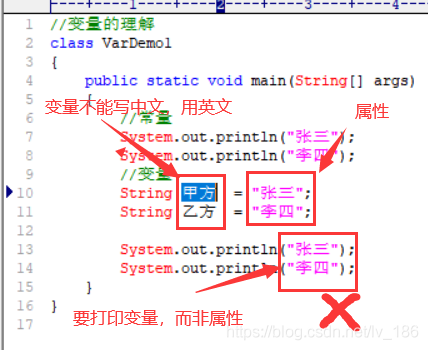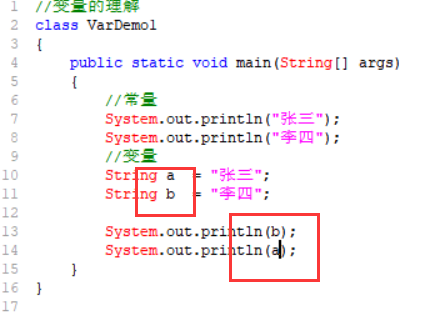• 一、 1、ABF 2、C 3、ABD 4、 B 5、 AC 6、 D 7、 D 8、D 9、C 10、AD 11、CD 二、 1、 package homework_0803; public class FloatDemo { ... // 定义一个float类型的变量f1，值为98.4 ... //定义一个float类型的变量
一、
1、ABF
2、C
3、ABD
4、 B
5、 AC
6、 D
7、 D
8、D
9、C
10、AD
11、CD
二、
1、
package homework_0803;

public class FloatDemo {
public static void main(String[] args) {
// 定义一个float类型的变量f1，值为98.4
float f1 = (float)98.4;
System.out.println(f1);
//定义一个float类型的变量f2，将f1的值赋值给f2
float f2 = f1;
System.out.println(f2);
//定义一个整型变量n，值为55
int n = 55;
System.out.println(n);
//定义一个double类型的变量d1，值为555.3
double d1 = 555.2;
System.out.println(d1);
//将n的值赋值给d1
d1 = n;  //自动转换
System.out.println(d1);
}
}


2、
package homework_0803;

public class CharTest {
public static void main(String[] args) {
// 定义字符变量c，并赋值为'S'
char c = 'S';
//输出c的值
System.out.println(c);
//定义字符变量c1，并赋值为78
char c1 = 78;
//输出c1的值
System.out.println(c1);

}

}


3、
package homework_0803;

public class StringDemo {
public static void main(String[] args) {
// 定义一个布尔类型的变量flag，赋值为true，并输出
boolean flag = true;
System.out.println(flag);
//定义一个字符串类型的变量str，值为"hello world"，并输出
String str = "hello world";
System.out.println(str);
//定义一个字符串类型的变量str1，值为空串
String str1 = "";
System.out.println(str1);
//将str的值赋值给str1，并输出
str1 = str;
System.out.println(str1);
}
}


4、
package homework_0803;

public class TypeExchange {
public static void main(String[] args) {
// 定义一个整型变量n，值为98
int n = 98;
System.out.println(n);
//定义一个char类型的变量ch，将变量n赋值给ch，然后输出ch的值
char ch = (char)n;
//因为int类型取值范围比char的取值范围大所以需要强转
System.out.println(ch);
//定义一个长整型变量var，值为190000000，并输出
long var = 190000000;//int类型转long类型，自动转换
System.out.println(var);
//定义一个double类型的变量d，将变量var的值赋值给d
double d = var;
System.out.println(d);
}
}


5、
package homework_0803;

public class TypeExchange2 {
public static void main(String[] args) {
// 将一个整型字面值67832赋值给char类型变量c
char c = (char)67832;
//并将c的值输出
System.out.println(c);
//定义一个整型变量n，值为65
int n = 65;
//定义一个字符型变量c1，赋值为n，并输出c1的值
char c1 =(char)n;
System.out.println(c1);
//定义一个长整型变量l，值为987654321
long l = 987654321;
//定义一个整型变量i，赋值为l，并输出i的值
int i =(int)l;
System.out.println(i);
//定义一个float类型变量f，将变量l的值赋值给f，并输出f的值
float f = l;
System.out.println(f);
//将float的值f，重新赋值给变量l，并输出l的值
l = (long)f;
System.out.println(l);
}
}




展开全文• JAVA变量和运算符作业 题目： 1．在JAVA语言中，下列能够作为变量名的是【 】。 A) if B) 3ab C) a_3b D) a-bc 2．在JAVA语言中，转义字符不可以表示【 】。 A) 任何字符 B)字符串 C)字母 D)小数点 3．表达式5...
JAVA-变量和运算符作业
题目：
1．在JAVA语言中，下列能够作为变量名的是【 】。
A) if     B) 3ab    C) a_3b    D) a-bc
2．在JAVA语言中，转义字符不可以表示【 】。
A) 任何字符   B)字符串   C)字母    D)小数点
3．表达式5/2+5%2-1的值是【  】。
A) 4     B) 2     C) 2.5    D) 3.5
4．下列数值类型的数据精度最高的是【  】。
A) int    B) float    C) double D) long
5．在JAVA语言中，下面的运算符中，优先级最高的是【  】。
A) %    B) ++    C) *=    D) >
6．能正确表示逻辑关系“a≥10 或 a≤0”的JAVA语言表达式是【  】。
A) a>=10 or a<=0    B) a>=10 | a<0    C) a>=10 && a<=0     D) a>=10 || a<=0
7．以下程序的输出结果是【   】。
public static void main(string[] args)
{
int a=5,b=4,c=6,d;
System.out.println(d=a>b?(a>c?a:c):b);
}
A) 5     B) 4     C) 6     D) 不确定
8．要使用变量score来存储学生某一门课程的成绩（百分制，可能出现小数部分），则最好将其定义为【 】类型的变量。
A) int    B) decimal   C) float    D) double
9、下列类型转换中正确的是【 】
(A) int i=’A’     (B) long L=1.1f   © int i=(float)1.1   (D) int i=1.1
10、以下选项中，合法的赋值语句是（）
(A) int a==1          (B) int i=1;int j=i++;  © int a=a+1=2;       (D) int i=int (j);
11、下列运算符中属于关系运算符的是（）
(A) ==    (B) =     © +=    (D) -=
12、阅读下面的程序，程序的运行结果为（）
int a=10,b=4,c=20,d=6;
int result=a++b+c–d;
(A)144
(B)160
©140
(D)164
13、阅读下面的程序，程序的运行结果为（）
int x=3,y=4,z=5;
string s=”xyz”
string result=s+x+y+z;
(A)xyz12 (B)xyz345 ©xyzxyz (D)12xyz
14、阅读下面的程序，程序的运行结果为（）
int i=10,j=3;
float m=213.5f,n=4.0f;
string str1=i%j+””;
string str2=m%n+””;
(A)1.0和1.5 (B)1和1.5 ©1.0和2.5 (D)1和2.5
答案：
1-5：CBBCB
6-10：DCCAB
11-14：ACBB


展开全文编程语言
• 1. 下列属于合法的 Java 标识符是？ABF A. $value B. Void C. class D. 1abc E. my value F.void_class 2. 下列哪一个选项不是Java的关键字？C A. class B. package C. Void D. static 3. 下列可以表示数值型数据... 一、选择 1. 下列属于合法的 Java 标识符是？ABF A.$value B. Void C. class D. 1abc E. my value F.void_class

2. 下列哪一个选项不是Java的关键字？C
A. class B. package C. Void D. static

3. 下列可以表示数值型数据的数据类型是？ABD
A. byte B. float C. boolean D. long

4. 关于数据类型的说法错误的是？B
A. 数据类型分为基本数据类型和引用数据类型
B. 数组属于基本数据类型
C. 类属于引用数据类型
D. int和double属于基本数据类型

5. 下列关于赋值运算符的说法正确的是？AC
A. 使用“=”给变量进行赋值
B. 使用“==”给变量进行赋值
C. "="叫作赋值运算符，将运算符右边的值赋给左边的变量
D. "="叫作赋值运算符，将运算符左边的值赋给右边的变量

6. 以下对double类型字面值的表示错误的是？D
A. 1.23 B. 1.23d C. 1.23D D.1.23f

7. 以下语句哪个是错误的？D
A. double d=12.3d; B. double d=12.3D; C. float f=1.23f; D.float f=1.23;

8. 下面代码运行后的结果为?  D
public class CharTest {
public static void main(String[] args) {
char c = 'A';
System.out.println(c);
char c1 = 65;
System.out.println(c1);
}
}

A. A B. 65 C. A 65 D.A A

9. 以下字符型字面值中，不合法的是? C
A. '@' B. '&' C. "M" D.'课'

10. 以下哪两个是合法的字符串字面值？AD
A. "" B. 'ab' C. 'a' D."\u0067"

11. 以下哪两条语句是正确的？CD
A. char ch=65539; B. int n=5.6f; C. double d=1.2f; D. double d=1.23;

二、编程
1. 分别定义float、double、int类型的数据，并打印输出。
public class FloatDemo {
public static void main(String[] args) {
float f1 = 98.4F;// 定义一个float类型的变量f1，值为98.4
float f2 = f1;//定义一个float类型的变量f2，将f1的值赋值给f2
int n = 55;//定义一个整型变量n，值为55
double d1 = 555.3;//定义一个double类型的变量d1，值为555.3
d1 = n;//将n的值赋值给d1
}
}

2. 定义字符型变量，赋值后输出。
public class CharTest {
public static void main(String[] args) {
char c = 'S';// 定义字符变量c，并赋值为'S'
System.out.println(c);// S
char c1 = 78;//定义字符变量c1，并赋值为78
System.out.println(c1);// N
}
}

3. 定义布尔类型和字符串类型的变量，为它们赋值，并打印输出。
public class StringDemo {
public static void main(String[] args) {
boolean flag = true;// 定义一个布尔类型的变量flag，赋值为true，并输出
System.out.println(flag);
String str = "hello world";// //定义一个字符串类型的变量str，值为"hello world"，并输出
System.out.println(str);
String str1 = "";//定义一个字符串类型的变量str1，值为空串
str1 = str;
System.out.println(str1);
}
}

4. 定义各种数据类型的变量，为它们赋值，并打印输出。
public class TypeExchange {
public static void main(String[] args) {
int n = 98;// 定义一个整型变量n，值为98
char ch;
ch = (char)n;
System.out.println(ch);//  b  定义一个char类型的变量ch，将变量n赋值给ch，然后输出ch的值
long var = 190000000;
System.out.println(var);//定义一个长整型变量var，值为190000000，并输出
double d;
d = var;//定义一个double类型的变量d，将变量var的值赋值给d
}
}

5. 定义各种数据类型的变量，按要求为它们赋值，并打印输出。
public class TypeExchange {
public static void main(String[] args) {
int x = 67832;
char c = (char)x;// 将一个整型字面值67832赋值给char类型变量c
System.out.println(c);//并将c的值输出
int n = 65;//定义一个整型变量n，值为65
char c1 = (char)n;
System.out.println(c1);// A 定义一个字符型变量c1，赋值为n，并输出c1的值
long l = 987654321L;//定义一个长整型变量l，值为987654321
int i = (int)l;
System.out.println(i);// 987654321 定义一个整型变量i，赋值为l，并输出i的值
float f = l;
System.out.println(f);// 9.8765434E8 定义一个float类型变量f，将变量l的值赋值给f，并输出f的值
l = (long)f;
System.out.println(l); //将float的值f，重新赋值给变量l，并输出l的值
}
}



展开全文• 变量初学错误 图片: ] 运行结果
变量初学错误
图片:]
运行结果展开全文• 1.指出下面程序中的编译错误，并更正 int lenght=10; System.out.println(length); 2.指出下面程序中的编译错误，并更正 ...关注公众号，回复"java变量"即可获取学习视频
• 1.下列属于合法的 Java 标识符是？（多选）ABF A. $value B. Void C. class D. 1abc E. my value F.void_class 2.下列哪一个选项不是Java的关键字？C A. class B. package C. Void D. static 3.下列可以表示数值型... • 1、上节课作业评讲总结 a)拼接一般步骤：先将字符串和变量一一排好，中间留空格，字符串该加转义符的加，最后在空格中间加连接符+ b)当两行内容要写在一句控制台语句输出里，在字符串最后的换行转义符后面要加连接... • 1、变量 变量的作用就是用来接收，保存，传递，操作数据 使用规则： 先声明，在赋值，在使用 变量的种类： 类型划分 基本类型变量(byte short int long float double char boolean) 引用类型变量(类类型，... • 下列属于合法的 Java 标识符是？（多选） A.$value B. Void C. class D. 1abc E. my value F.void_class 选择：ABF 下列哪一个选项不是Java的关键字？ A. class B. package C. Void D. static 选择：C 下列可以...
• 我是小康小白，一个平平无奇的Java，Python小白。热爱有趣的文字，生活和远方。 个人博客：https://blog.csdn.net/weixin_45791445 有问题欢迎QQ联系：1059320343 （记得备注CSDN） 个人微信公众号：小康小白 一，...字符串 编程语言
• java继承作业如何插入一段漂亮的代码片 今天做的java继承作业，日常记录。 题目如下： 定义父类Person，包含身份证号id，姓名name成员变量，同时包含两个构造方法： 一个带参数的，为对象各个成员变量赋值，另一个是...
• 一、windows10配置java环境 下载 JDK 下载地址：https://www.oracle.com/technetwork/java/javase/downloads/index-jsp-138363.html ...2. 新建JAVA_HOME 变量 点击 New（新建）… 按钮 输入: 变量名：J
• 声明变量存储商品信息（商品名称、商品价格和商品库存数量）。 输出商品信息。 实现思路： 打印商品商品信息实现步骤： 声明变量存储商品信息。为变量赋值。 输出变量的值。 运行程序。 ...
• 下列属于合法的 Java 标识符是？（A B F） A. $value B. Void C. class D. 1abc E. my value F.void_class 标识符的解析： 标识符由数字（0～9）、美元符号（$）、下划线（_）以及Unicode字符集组合构成。 标识符各...
• 声明变量存储个人信息（姓名、年龄、性别、地址以及余额），通过键盘输入个人信息并存储在相应的变量中， 最后将个人信息输出。 实现思路： 声明存储姓名、年龄、性别、地址以及账户余额的变量。 定义 ...
• 1.下列属于合法的Java标识符是?(多选) A.\$valueB.VoldC.classD.1abcE.myvalueF.void_class ABF 2.下列哪一一个选项不是Java的关键字? A.classB.packageC.VoidD.static C 3.下列可以表示数值型数据的数据类型是?(多选...
• JAVA自学作业02 1、什么是标识符?由哪些部分组成?常见的命名规则有哪些? 标识符是用户为变量的内存空间所定义的字符序列； 可以由字母、下划线、美元符号以及数字组成，但数字不可作为首字符。标识符不可与 JAVA中...
• 课上问题 1.早期我们经常这样定义变量 int value=100; 前面的示例中这样定义变量 ...2.对于原始数据类型的变量（比如int)，可以直接使用“==”判断两变量值是否相等，对象变量也可以使用“==......

# java变量作业java 订阅